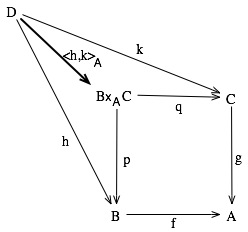# Categorical Equalizers

Category theory is really all about building math using composition. Everything we do, we do it by defining things completely in terms of composition. We’ve seen a lot of that. For example, we defined subclasses (and other sub-things) in terms of composition. It sounds strange, but it took me an amazingly long time to really grasp that. (I learned category theory from some not-very-good textbooks, which were so concerned with the formalisms that they never bothered to explain why any of it mattered, or to build any intuition.)

One thing that’s really important that we haven’t talked about yet is equality. In category theory, we define equality on arrows using something called a pullback. We’ll use that notion of equality for a lot of other things – like natural transformations. But before we can do pullbacks, we need to look at a weaker notion of arrow equality – something called the equalizer of a pair of arrows.

As part of my attempt to be better than those books that I complained about, we’ll start with intuition, by looking at what an equalizer is in terms of sets. Suppose we have two functions$f, g : A \rightarrow B$.

Now, in addition, suppose that they have a not-empty intersection: that is, that there’s some set of values$x \in A$ for which$f(x) == g(x)$. We can take that set of values, and call it$C$.$C$ is the equalizer of the functions$f$ and$g$. It’s the largest set$C$ where if you restrict the inputs to the functions
to members of$C$, then$f$ and$g$ are equal.

Now, let’s look at the category theoretic version of that. We have objects$A$ and$B$. We have two arrows$f, g : A \rightarrow B$. This is the category analogue of the setup of sets and functions from above.

To get to the equalizer, we need to add an object C which is a subobject of A (which corresponds to the subset of A on which f and g agree in the set model).

The equalizer of$A$ and$B$ is the pair of the object$C$, and an arrow$i : C \rightarrow A$. (That is, the object and arrow that define C as a subobject of A.) This object and arrow must satisfy the following conditions:

1.$f \circ i = g \circ i$
2.$(\forall j: D \rightarrow A)$ if$f \circ j = g \circ j$ then$(\exists 1 k : D \rightarrow C)$ such that$i \circ k = j$

That second one is the mouthful. What it says is:

• Suppose that I have any arrow j from some other object D to A:
• if f and g agree on composition about j, then there can only be one unique arrow from C to D which composes with j to get to A.

In other words, (C, i) is a selector for the arrows on which A and B agree; you can only compose an arrow to A in a way that will compose equivalently with f and g to B if you go through (C, i).

Or in diagram form, k in the following diagram is necessarily unique:There are a couple of interesting properties of equalizers that are worth mentioning. The morphism in an equalizer is a always monic arrow (monomorphism); and if it’s epic (an epimorphism), then it must also be iso (an isomorphism).

The pullback is very nearly the same construction as the equalizer we just looked at; except it’s abstracting one step further.

Suppose we have two arrows pointing to the same target:$f : B \rightarrow A$ and$g : C \rightarrow A$. Then the pullback of of f and g is the triple of an object and two arrows$(B \times_A C, p : B \times_A C \rightarrow B, q : B\times_A C \rightarrow C)$. The elements of this triple must meet the following requirements:

1.$f \circ p = g \circ q$
2.$(f \circ p) : B\times_A C \rightarrow A$
3. for every triple$(D, h : D \rightarrow B , k : D \rightarrow C)$, there is exactly one unique arrow$\langle h,k \rangle_A : D \rightarrow B \times_A C$ where$p \circ \langle h,k \rangle_A = h$, and$q \circ \langle h,k \rangle_A = k$.As happens so frequently in category theory, this is clearer using a diagram.If you look at this, you should definitely be able to see how this corresponds to the categorical equalizer. If you’re careful and clever, you can also see the resemblance to categorical product (which is why we use the ×A syntax). It’s a general construction that says that f and g are equivalent with respect to the product-like object B×AC.

Here’s the neat thing. Work backwards through this abstraction process to figure out what this construction means if objects are sets and arrows are functions, and what’s the pullback of the sets A and B?${ (x,y) \in A \times B : f(x) = g(y) }$

Right back where we started, almost. The pullback is an equalizer; working it back shows that.